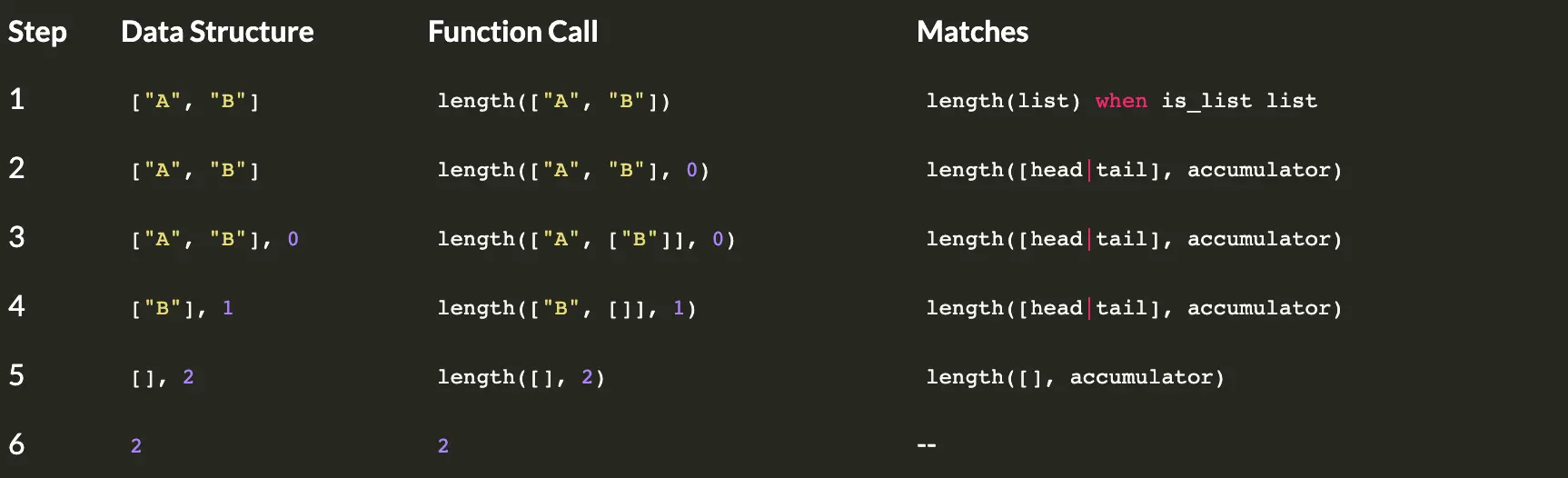# Recursion and Pattern Matching in Elixir

In this post I will try and explain how pattern matching and recursion work in Elixir with some (hopefully) simple examples.

I’m not going go into the ‘why’, for that, there are many people better qualified than me to explain.

What I hope to acheive is a mental model for reasoning about what’s actually happening.

# Let’s start at the very beginning

Imagine we have a list of things and we want to know the length of it. The simplest case would be a list that is empty.

So given this list `[]`, we would expect the length to be `0`

Let’s write a module that deals with this.

``````defmodule MyEnumerator do
def length([]), do: 0
end
``````

What we have here is a function that will accept an empty list and return 0.

If we call it with anything other than that, we will see an error.

``````iex(2)> MyEnumerator.length([])
0
iex(3)> MyEnumerator.length(["A", "B", "C"])
** (FunctionClauseError) no function clause matching in MyEnumerator.length/1
iex:2: MyEnumerator.length(["A", "B", "C"])
``````

OK, this is definitely a success, we can now determine that an empty list has a length of 0. Well done!

# And for our next trick

What about a list that has one element? Or a list that has a thousand?

Now we need to actually figure out what to do with the list to determine its length. One way is to use an accumulator. An accumulator is a place we can store the current length of the list, we can then go through and add 1 for every element.

Before we do that, let’s do the simplest thing that could work.

``````defmodule MyEnumerator do
def length([]), do: 0
def length([_]), do: 1
def length([_, _]), do: 2
end
``````

We can now handle a case where the list has one or two elements.

``````iex(4)> MyEnumerator.length(["A"])
1
iex(5)> MyEnumerator.length(["A", "B"])
2
iex(6)> MyEnumerator.length(["A", "B", "C"])
** (FunctionClauseError) no function clause matching in MyEnumerator.length/1
iex:9: MyEnumerator.length(["A", "B", "C"])
``````

Now we can handle lists of length 1 and 2! Huzzah.

And we could, go on like this forever, but that’s gonna get tedious really quickly!

# Recursion to the rescue

Here we’re going to start breaking our brain a little if we’ve come from other programming paradigms. It’s OK, it’ll be worth it, I promise (no guarantees though 😜).

So now we need to be able to match a list of any length.

``````defmodule MyEnumerator do
def length([]), do: 0

def length(list) when is_list(list) do
length(list, 0)
end
end
``````

Here we will have a function that will accept one parameter, which is a list. I’ve put the guard clause here because we only care about lists.

This function immediately calls another function, which take two parameters.

We haven’t defined this yet.

This may look a bit funky, but I’ll try and explain what’s happening here soon.

``````defmodule MyEnumerator do
def length([]), do: 0

def length(list) when is_list(list) do
length(list, 0)
end

def length([], accumulator), do: accumulator

def length([_head | tail], accumulator) do
length(tail, accumulator + 1)
end
end
``````

We now have two functions, in the first we’re saying, if you give me an empty list AND and an accumulator, just return the accumulator. This is also known as a base clause, this is where the recursion stops. Makes sense, right? Once a list is empty, we can stop counting the elements it contains.

If the function receives a list with 1 or more elements, we’ll start/continue counting the elements by adding one to the accumulator and then calling length with the tail of the list. This is recursion.

Let’s try and work through a short list to visualise how the functions are called and the data structures passed around.Hopfully you can see that this has a very similar effect to ‘popping’ from a stack in another paradigm, but the nice thing here is our orginal list remains unchanged.

It’s kinda neat though, that we just keep calling the same named function until such time as we’re done.

This ends my simple intro to recursion, for something a bit more in depth, I recommend http://learnyousomeerlang.com/recursion#hello-recursion

# Pattern matching

We’ve had a basic introduction to recursion, which relied heavily on pattern matching too.

Pattern matching can be a great way to reduce conditional logic, allowing very clear isolation and simpler testing.

A common coding challenge is to write code that determines whether a given year is a leap year.

The rules are fairly simple. A leap year is evenly divisible by 4, except if years what are evenly divisible by 100, unless it is also evenly divisible by 400!.

So we could do the super terse thing and make just a bunch of if `rem(year, 4) == 0 || ...`

Or we could make use of pattern matching.

``````defmodule Year do
def is_leap?(year) do
case {rem(year, 4), rem(year, 100), rem(year, 400)} do
{0, 0, 0} -> true
{0, 0, _} -> false
{0, _, 0} -> true
_ -> false
end
end
end
``````

With this style, we can make decisions based on the shape or pattern of our data.

Now this example isn’t necessarily the best use case for pattern matching, but I think it highlights some of the potentional.

When you combine pattern matching with the pipeline operator and multiple function definitions we have a really nice opportunity to get rid of nested and hard to reason conditional logic.